# Students

If the fifth-grade class left 20% of students and in the seventh grade were added two pupils, in the eighth one pupil, the number has not changed in the ninth grade, but it is now tenth students less than it was in the fifth grade.

How many pupils are in the 9th grade now?

d =  27

### Step-by-step explanation:

b=a - 0.20 a
c = 2+b
d = c+1
d = a - 0.10a

b=a - 0.20•a
c = 2+b
d = c+1
d = a - 0.10•a

0.8a-b = 0
b-c = -2
c-d = -1
0.9a-d = 0

a = 30
b = 24
c = 26
d = 27

Our linear equations calculator calculates it.Did you find an error or inaccuracy? Feel free to write us. Thank you!

Showing 1 comment:Math student
someone do it!Tips to related online calculators
Do you have a linear equation or system of equations and looking for its solution? Or do you have a quadratic equation?

## Related math problems and questions:

• Unions now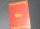The plant has 1,250 employees. The Trade Unions (former ROH, OH) is 18% male, 23% female. Plant management reported that in the trade union is 20% of all employees. How many men and women are in the plant, and how many are men and women in trade unions?
• Columns of two and threeWhen students in one class stand in columns of two, there is none left. When he stands in columns of three, there is one student left. There are 5 more double columns than three columns. How many students are in the class?
• Mini-surveyIn the mini-survey of our class about the popularity of individual subjects, it turned out that 11.1% of pupils like mathematics, 18.5% are enjoying languages, 30.4% of pupils like physical education, and the remaining 12 pupils have several popular subje
• Prospering studentsThere are girls and 30 boys in the class. Boys prospering 28, girls all. How many girls are in the class and how many boys are there, if all the prospering students are 95%?
• Jane classWhen asked how many students are in class, Jane said, if we increase the number of students in our class by a hundred % and then add half the number of students, we get 100. How many students are in Jane's class?
• Lookout towerHow high is the lookout tower? If each step was 3 cm lower, 60 more of them were on the lookout tower. If it were 3 cm higher again, it would be 40 less than it is now.
• Three piles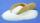100 kg of sugar we divide into three piles. The first pile is small. If we added to the second 2 kg of sugar, it would have 25% more sugar than the first pile. If we add 3 kg of sugar to the third pile it would have 20% more sugar than the 2nd pile. How m
• Crowns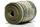1180 crowns are divided into three people: A got 20% less than B and C by 15% more than B. How many got A, B, and C?
• MerchantThe merchant lower cost by 10% in December when it was not sold. Again in January lower cost by 20% and now costs 576 €. A. How much did the goods stand originally? B, how much cost the goods after the first lowering? C, How many percents total merchant l
• Four numbersThe first number is 50% second, the second number is 40% third, the third number is 20% of the fourth. The sum is 396. What are the numbers?
• Two math problems1) The sum of twice a number and -6 is nine more than the opposite of that number. Find the number. 2) A collection of 27 coins, all nickels, and dimes, is worth \$2.10. How many of each coin are there? The dime, in United States usage, is a ten-cent coin.
• Dividing moneyThomas, Honza and Vasek are to divide 1220kč. Honza got 25% more than Thomas. Vasek 20% less than Thomas. How CZK each got?
• 9.A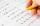9.A to attend more than 20 students but fewer than 40 students. A third of the pupils wrote a math test to mark 1, the sixth to mark 2, the ninth to mark 3. No one gets mark 4. How many students of class 9.A wrote a test to mark 5?
• In the classroomThere are 30 boys and a few girls in the class. In the six months, 28 boys and all girls benefited, which was 95% of all pupils. How many pupils are there in the classroom?
• 25 pupils25 pupils attend the school dance group, which is 5 percent of all pupils in the school. The judo class is attended by 20 pupils of the school, a quarter of whom also go to a dance group. How many schoolchildren do not go to a dance or judo group?
• Cross-country competition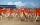Cross-country competition was attended by 76% of pupils of class 9.B. Class consis of 16 boys, two of whom did not run and 4 girls did not run. How many girls go to class 9.B?
• Sum of three numbersThe sum of three numbers from which the second number is 20% smaller than the first number and the third number is 25% smaller than the second number is 96. Determine these numbers.